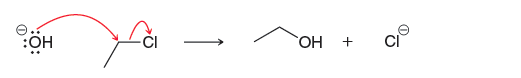# Problem: Consider the following reaction: This reaction has been determined to be second order. How will the rate be affected if the concentration of chloroethane is tripled?

###### Problem Details

Consider the following reaction: This reaction has been determined to be second order.

How will the rate be affected if the concentration of chloroethane is tripled?What scientific concept do you need to know in order to solve this problem?

Our tutors have indicated that to solve this problem you will need to apply the Kinetics concept. If you need more Kinetics practice, you can also practice Kinetics practice problems.

What is the difficulty of this problem?

Our tutors rated the difficulty ofConsider the following reaction: This reaction has been dete...as medium difficulty.

How long does this problem take to solve?

Our expert Organic tutor, Chris took undefined 53 seconds to solve this problem. You can follow their steps in the video explanation above.

What textbook is this problem found in?

Our data indicates that this problem or a close variation was asked in Organic Chemistry - Klein 1st Edition. You can also practice Organic Chemistry - Klein 1st Edition practice problems.